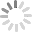# How to Find Duplicates in Excel - Easy Excel Tutorial

What are Excel formula and the difference between formula and function in Excel? Formula is used to calculate value of the cell and Functions are predefined formulas that are already present in Excel. For taking more benefits from Excel Formulas we can add manually, multiply, subtract values, etc. Excel Formulas actually makes our work easier. In short, we can say that Formula is a user defined calculation While Function is a built-in calculation. We can use a single function if we are using a formula. Do you want to know how to find duplicates in Excel? If yes, then read content below.

## Option 1: How to Find Case-sensitive Duplicates in Excel

Simple formula to perform a case sensitive match:

`{=MATCH (TRUE, EXACT(range,value),0)}`

For a case sensitive match, Exact function with Match is used in the formula. Let's have a look at an example below.

`{=MATCH(TRUE,EXACT(B5:B11,E6),0)}`

How this formula works? MATCH function is not case sensitive, so the following formula returns 1.

`=MATCH("JAMES",B5:B11,0)`

To add Case Sensitivity we use the Exact function like this:

`EXACT(B5:B11,E6)`

## Option 2: How to Find Duplicate Rows in Excel

Let's have a look below to Excel find duplicate rows. Formula used to find duplicate rows:

`=COUNTIFS(\$A\$2:\$A\$8, \$A2, \$B\$2:\$B\$8, \$B2) `

We will need to use the COUNTIFS function instead of COUNTIF to find duplicate rows. Look in the picture below:## Option 3: How to Find Duplicate Values in 2 Columns in Excel

We have already found duplicate rows in Excel now let's have a look with us to find duplicates in two columns in Excel.

Gerneric Formula: `=AND(COUNTIF(range1,A1),COUNTIF(range2,A1))`Use this formula to find duplicate values in two columns in Excel we use a conditional formatting with a formula based on COUNTIF and AND function.

`=AND(COUNTIF(range1,B5),COUNTIF(range2,B5))`

When rule created both ranges need to create at the same time. Use this formula to find duplicates in two columns.

## Option 4: Formula to Find Duplicates in Excel

Formula to find duplicates in Excel is no more difficult now.

• Step 1: On the Home tab, in the Styles group, choose Conditional Formatting.• Step 2: Now you need to choose Highlight Cells Rules and then choose Duplicate Values.• Step 3: Now you need to choose a formatting-style.• Step 4. It's end! Excel highlights the duplicate names.## Option 5: Other Ways to Deal with Duplicates

We have already found Excel find duplicates in column, now we are going to highlight, remove, select, copy or move duplicates.

### Now we are going to highlight Duplicate values:

• Step 1: choose cell that you want to check for duplicates
• Step 2: Now you need to choose Home > Conditional Formatting > Highlight Cells Rules > Duplicate Values.
• Step 3:  In the field with Value with, you need to choose the formatting you want to apply to duplicates at the end choose OK.

In such a way duplicate values will be highlighted.

### Now we are going to remove Duplicate values:

• Step 1: Choose cell that has a duplicate value.
• Step 2: Now choose Data and then Remove Duplicates, Under Columns, check or uncheck the columns where you want to remove the duplicates.• Step 3: After uncheck or check the column, at the end choose OK.

### Now we are going to select Duplicate values:

If you want to select Duplicate value including column headers, filter them, and then choose any filtered cell to select it, at the end you need to press Ctrl + A.

### Now we are going to copy Duplicate values:

If you want to copy duplicates select them, press Ctrl + C, then open another sheet, choose the upper-left cell of the range where you want to copy the duplicates, and press Ctrl + V, to paste them.

### Now we are going to Move Duplicate values:

To move duplicates to another sheet, perform the same steps with the only difference that you press Ctrl + X (cut) instead of Ctrl + C (copy).

## Extra Tips: How to Recover Forgotten Excel Password

Now recovering forgotten Excel password is no more difficult. PassFab for Excel has made easier to recover lost Excel password within few minutes. This software is 100% secure and reliable to use. Here is the instruction to use this PassFab for Excel tool.

• Stage 1: Download PassFab for Excel and then install it. You will see the main interface. Choose "Recover Excel Open Password" feature.• Stage 2: If you have installed it then Choose Add button, import the file for which you are looking for the password.• Stage 3: Now three Attack Types will be available, which are Dictionary Attack, Brute-force Attack and Brute-force with Mask Attack.
•• Stage 4: After attack type selection, click "Recover" to complete the recovery process.• Stage 5: After few minutes, a pop message will appear on the screen that your password has been recovered.## Bottom Line